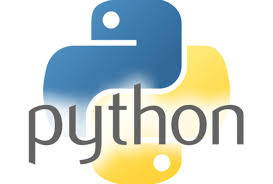21.What are tuples in Python?
A tuple is another sequence data type that is similar to the list. A tuple consists of a number of values separated by commas. Unlike lists, however, tuples are enclosed within parentheses.

22.What is the difference between tuples and lists in Python?
The main differences between lists and tuples are − Lists are enclosed in brackets ( [ ] ) and their elements and size can be changed, while tuples are enclosed in parentheses ( ( ) ) and cannot be updated. Tuples can be thought of as read-only lists.

23.What is the output of print tuple if tuple = ( ‘abcd’, 786 , 2.23, ‘john’, 70.2 )?
It will print complete tuple. Output would be (‘abcd’, 786, 2.23, ‘john’, 70.200000000000003).

24.What is the output of print tuple if tuple = ( ‘abcd’, 786 , 2.23, ‘john’, 70.2 )?
It will print first element of the tuple. Output would be abcd.

25.What is the output of print tuple[1:3] if tuple = ( ‘abcd’, 786 , 2.23, ‘john’, 70.2 )?
It will print elements starting from 2nd till 3rd. Output would be (786, 2.23).

26.What is the output of print tuple[2:] if tuple = ( ‘abcd’, 786 , 2.23, ‘john’, 70.2 )?
It will print elements starting from 3rd element. Output would be (2.23, ‘john’, 70.200000000000003).

27.What is the output of print tinytuple * 2 if tinytuple = (123, ‘john’)?
It will print tuple two times. Output would be (123, ‘john’, 123, ‘john’).

28.What is the output of print tuple + tinytuple if tuple = ( ‘abcd’, 786 , 2.23, ‘john’, 70.2 ) and tinytuple = (123, ‘john’)?
It will print concatenated tuples. Output would be (‘abcd’, 786, 2.23, ‘john’, 70.200000000000003, 123, ‘john’).

29.What are Python’s dictionaries?
Python’s dictionaries are kind of hash table type. They work like associative arrays or hashes found in Perl and consist of key-value pairs. A dictionary key can be almost any Python type, but are usually numbers or strings. Values, on the other hand, can be any arbitrary Python object.

30.How will you create a dictionary in python?
Dictionaries are enclosed by curly braces ({ }) and values can be assigned and accessed using square braces ([]).

dict = {}
dict[‘one’] = “This is one”
dict = “This is two”
tinydict = {‘name’: ‘john’,’code’:6734, ‘dept’: ‘sales’}